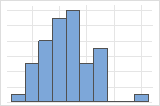# Interpret the key results for Bootstrapping for 2-sample means

Complete the following steps to interpret a 2-sample mean bootstrapping analysis. Key output includes the histogram, the average, and the confidence interval.

## Step 1: Examine the shape of your bootstrap distribution

Use the histogram to examine the shape of your bootstrap distribution. The bootstrap distribution is the distribution of the difference in means from each resample. The bootstrap distribution should appear to be normal. If the bootstrap distribution is non-normal, you cannot trust the results.The distribution is usually easier to determine with more resamples. For example, in these data, the distribution is ambiguous for 50 resamples. With 1000 resamples, the shape looks approximately normal.

## Step 2: Determine a confidence interval for the population mean

First, consider the difference in means from the bootstrap sample, and then examine the confidence interval.

The difference in means of the bootstrap sample is an estimate of the difference in population means. Because the difference of the bootstrap sample is based on sample data and not the entire population, it is unlikely that the difference of the bootstrap sample equals the difference in population means. To better estimate the difference in population means, use the confidence interval.

Confidence intervals are based on the sampling distribution of a statistic. If a statistic has no bias as an estimator of a parameter, its sampling distribution is centered at the true value of the parameter. A bootstrapping distribution approximates the sampling distribution of the statistic. Therefore, the middle 95% of values from the bootstrapping distribution provide a 95% confidence interval for the parameter. The confidence interval helps you assess the practical significance of your estimate for the population parameter. Use your specialized knowledge to determine whether the confidence interval includes values that have practical significance for your situation.

###### Note

Minitab does not calculate the confidence interval when the number of resamples is too small to obtain an accurate confidence interval.

By using this site you agree to the use of cookies for analytics and personalized content.  Read our policy